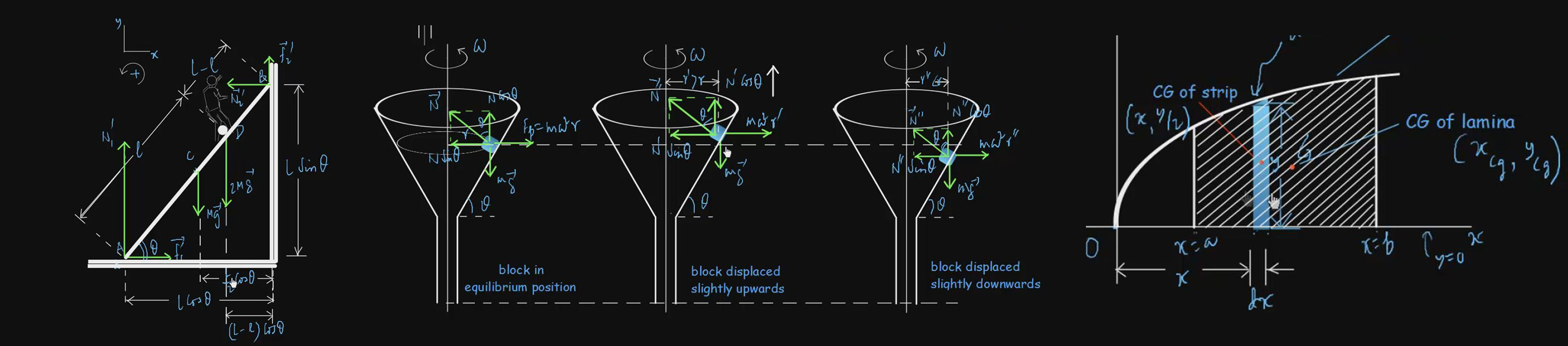# Gravitation

We told you in topic 4 that the gravitational force is one of the three fundamental forces of nature, the other two being the electromagnetic force and the nuclear force. The nuclear force operates inside an atomic nucleus and does not make its presence felt in everyday life. The electromagnetic force is often disguised as various forms of contact force. But whether it is the orbital motion of a planet round the sun or the free fall of an apple from a tree, the effect of the gravitational force is easy for all to feel. For your easy understanding of the subject and to increase your level of command to crack and solve the variety of numerical problems, we have video lectures on Newton’s Law of Universal Gravitation, Determination of the Gravitational Constant G, Kepler’s Laws of Planetary Motion, Gravity, Gravitational Field, Gravitational Potential Energy, Gravitational Potential, Escape Speed, Natural and Artificial Satellites of Motion, Mechanical Energy of Satellite-Earth System, etc.

#### Basic level videos

Determination Of Gravitational Constant G 38:51 Basic
200 3
Escape Speed 47:07 Basic
300 5
Gravitational Field And Potential Due To Some Extended Bodies 51:39 Basic
300 5
Gravitational Field And Potential Due To Some Extended Bodies II 39:09 Basic
200 3
Gravitational Field 45:32 Basic
250 7.5
Gravitational Potential Energy 1:00:14 Basic
300 5
Gravitational Potential 45:04 Basic
250 4
Gravity 47:34 Basic
300 5
Kepler's Laws Of Planetary Motion 48:45 Basic
300 5
Mechanical Energy Of Satellite-Earth System 1:02:11 Basic
350 7.5
More Problems On Escape Speed And Gravitational Potential 1:05:27 Basic
350 7.5
More Problems On Mechanical Energy Of Satellite-Earth System 52:36 Basic
300 5
Natural And Artificial Satellites Of Planets 54:26 Basic
300 5
Newton's Law Of Universal Gravitation 32:23 Basic
200 3
Problems On Escape Speed 53:31 Basic
300 5
Problems On Gravitational Field And Potential Due To Extended Bodies 1:11:17 Basic
350 7.5
Problems On Newton's Law Of Gravitation 1:05:22 Basic
350 7.5
Problems On Orbital Motion Of Satellites 01:07:09 Basic
350 7.5
Problems On Variation Of Acceleration Due To Gravity 1:00:27 Basic
300 5
Variation Of Acceleration Due To Gravity I 58:19 Basic
300 5
Variation Of Acceleration Due To Gravity II 57:07 Basic
300 7.5

#### Advanced level Videos Note: (CE) Stands for Problems from Competitive Examination Papers

Problems On Gravitation (CE) 1:11:03
350
7.5
Problems On Gravitation II (CE) 1:04:50
350
7.5
Problems On Gravitation III (CE) 1:22:47
350
7.5
Advanced Level Problems On Gravitation II 01:04:52
350
7.5
Advanced Level Problems On Gravitation I 1:10:40
350
7.5
Advanced Level Problems On Gravitation-III 01:19:03
350
7.5
Advanced Level Problems On Gravitation-IV 01:22:10
350
7.5
Advanced Level Problems On Gravitation V 01:03:44
350
7.5